# NCERT Solutions for Class 6 Maths Chapter 5 Understanding Elementary Shapes

Here you will get Chapter 5 Understanding Elementary Shapes Class 6 Maths NCERT Solutions which will help you in rectifying all those mistakes that can happen in the exams. You can download PDF of NCERT Solutions for Class 6 Maths Chapter 5 Understanding Elementary Shapes which are accurate and detailed are prepared by Studyrankers experts. It will serve as beneficial tool that can be used to recall various questions any time.

Chapter 5 NCERT Solutions will be helpful in completing your homework in less time. Through these NCERT Solutions. a student can figure out the latest marking scheme and prepare their answers accordingly.Page No: 88

Exercise 5.1

1. What is the disadvantage in comparing line segments by mere observation?

There may be chance of error due to improper viewing.

2. Why is it better to use a divider than a ruler, while measuring the length of a line segment?

It is better to use a divider than a ruler, because the thickness of the ruler may cause difficulties in reading off her length. However, divider gives up the accurate measurement.

3. Draw any line segment, say AB. Take any point C lying in between A and B. Measure the lengths of AB, BC and AC. Is AB = AC + CB?
[Note: If A, B, C are any three points on a line, such that AC + CB = AB, hence we can be sure that C lies between A and B.]

Yes.AB = 6.5 cm, AC = 3cm, CB = 3.5 cm
AC + CB = 3 cm + 3.5 cm = 6.5 cm = AB

4. If A, B, C are three points on a line such that AB = 5 cm, BC = 3cm and AC = 8 cm, which one of them lies between the other two?

AC is the longest line segment, thus B is the point between A and C.

5. Verify whether D is the mid-point of AG.AD = 3 units, DG = 3 units
Thus, D is the mid-point.6. If B is the mid-point of AC and C is the mid-point of BD, where A, B, C, D lie on a straight line, say why AB = CD?

B is the mid-point of AC.
∴ AB = BC ….(i)
And C is the mid-point of BD.
∴ BC = CD ….(ii)
From eq. (i) and (ii),
AB = CD
7. Draw five triangles and measure their sides. Check in each case, of the sum of the lengths of any two sides is always less than the third side.

Yes, the sum of two sides of a triangle is always greater than the third side.Apply Pythagorean theorem to find the hypotenuse i.e 14.14cm

Exercise 5.2

1. What fraction of a clockwise revolution does the hour hand of a clock turn through, when it goes from
(a) 3 to 9, (b) 4 to 7, (c) 7 to 10, (d) 12 to 9, (e) 1 to 10, (f) 6 to 3

(a) 1/2 or two right angles
(b) 1/4 or one right angle
(c) 1/4 or one right angle
(d) 3/4 or three right angles.
(e) 3/4 or three right angles.
(f) 3/4 or three right angles.

2. Where will the hand of a clock stop if it:
(a)starts at 12 and make 1/2 of a revolution, clockwise?
(b)starts at 2 and makes 1/2 of a revolution, clockwise?
(c)starts at 5 and makes 1/4 of a revolution, clockwise?
(d)starts at 5 and makes 3/4 of a revolution, clockwise?

(a) At 6,
(b) At 8,
(c) At 8,
(d) At 2

3. Which direction will you face if you start facing:(a) East and make 1/2 of a revolution clockwise?
(b) East and make 1.1/2 of a revolution clockwise?
(c) West and makes 3/4 of a revolution, clockwise?
(d) South and make one full revolution?
(Should we specify clockwise or anti-clockwise for this last question? Why not?)

(a) West,
(b) West ,
(c) North,
(d) South
(For answer (d), it is immaterial whether we turn clockwise or anticlockwise because one full revolution will bring us back to the original position)

4. What part of a revolution have you turned through if you stand facing:
(a) East and turn clockwise to face north?
(b) South and turn clockwise to face east?
(c) West and turn clockwise to face east?

(a) 3/4
(b) 3/4
(c) 1/2

5. Find the number of right angles turned through by the hour hand of a clock when it goes from:
(a) 3 to 6, (b) 2 to 8, (c) 5 to 11, (d) 10 to 1, (e) 12 to 9, (f) 12 to 6

(a) One right angle
(b) Two right angles
(c) Two right angles
(d) One right angle
(e) Three right angles
(f) Two right angles

6. How many right angles do you make if you start facing:
(a) South and turn clockwise to west?
(b) North and turn anti-clockwise to east?
(c) West and turn to west?
(d) South and turn to north?

(a) One right angle
(b) Three right angles
(c) Four right angles
(d) Two right angles

7. Where will the hour hand of a clock stop if it starts:
(a) from 6 and turns through 1 right angle?
(b) from 8 and turns through 2 right angles?
(c) from 10 and turns through 3 right angles?
(d) from 7 and turns through 2 straight angles?

(a) At 9,
(b) At 2,
(c) At 7,
(d) At 7

Exercise 5.3

1. Match the following:
 (i) Straight angle (a) less than one-fourth a revolution (ii) Right angle (b) more than half a revolution (iii) Acute angle (c) half of a revolution (iv) Obtuse angle (d) one-fourth a revolution (v) Reflex angle (e) between 1414 and 1212 of a revolution (f) one complete revolution

(i) → (c)
(ii) → (d)
(iii) → (a)
(iv) → (e)
(v) → (b)

2. Classify each one of the following angles as right, straight, acute, obtuse or reflex:(a) Acute angle
(b) Obtuse angle
(c) Right angle
(d) Reflex angle
(e) Straight angle
(f) Acute angle

Exercise 5.4

1. What is the measure of (i) a right angle? (ii) a straight angle?

(i) The measure of a right angle is 90°
(ii) The measure of a straight angle is 180°

2. Say True or False:
(a) The measure of an acute angle < 90°
(b) The measure of an obtuse angle <90°
(c) The measure of a reflex angle > 180°
(d) The measure of °ne complete revolution= 360°
(e) If m∠A = 53° and m∠B = 35° then m∠A > m∠B.

(a) True,
(b) False,
(c) True,
(d) True,

(e) True

3. Write down the measure of:
(a) some acute angles
(b) some obtuse angles
(give at least two examples of each)

(a) Acute angles measure less than 90° e.g. 54°,76°
(b) Obstuse angles measure greater than 90°. e.g. 105°, 154°

4. Measure the angles given below, using the protractor and write down the measure:

(a) 40°
(b) 130°
(c) 90°
(d) 60°
Other 2 angles i.e 90 degrees and 120 degrees

5. Which angle has a large measure? First estimate and then measure:
Measure of angle A =
Measure of angle B =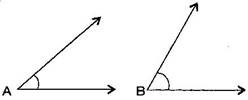∠B has a larger measure.
∠A = 40° and ∠B = 65°

6. From these two angles which have larger measure? Estimate and then confirm by measuring them: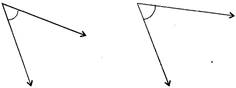The Second angle has a larger measure.

7. Fill in the blanks with acute, obtuse, right or straight:
(a) An angle whose measure is less than that of a right angle is ________________.
(b) An angle whose measure is greater than that of a right angle is ________________.
(c) An angle whose measure is the sum of the measures of two right angles is _________.
(d) When the sum of the measures of two angles is that of a right angle, then each one of them is ________________.
(e) When the sum of the measures of two angles is that of a straight angle and if one of them is acute then the other should be ________________.

(a) acute angle,
(b) obtuse angle,
(c) straight angle,
(d) acute angle,

(e) obtuse angle

8. Find the measure of the angle shown in each figure. (First estimate with your eyes and then find the actual measure with a protractor).(i) 30° (ii) 120° (iii) 60° (iv) 150°

9. Find the angle measure between the hands of the clock in each figure: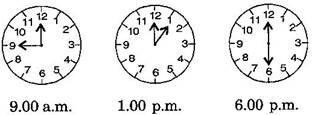(i) 90° (Right angle)
(ii) 30° (Acute angle)
(iii) 180° (Straight angle)

10. Investigate:
In the given figure, the angle measure 30°. Look at the same figure through a magnifying glass. Does the angle become larger? Does the size of the angle change?No, the measure of angle will be same.

11. Measure and classify each angle: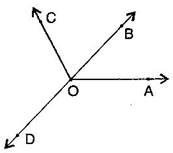Angle ∠AOB ∠AOC ∠BOC ∠DOC ∠DOA ∠DOB Measure Type

 Angle ∠AOB ∠AOC ∠BOC ∠DOC ∠DOA ∠DOB Measure 40° 130° 90° 90° 140° 180° Type Acute Obtuse Right Right Obtuse straight

Exercise 5.5

1. Which of the following are models for perpendicular lines:
(a)The adjacent edges of a table top.
(b)The lines of a railway track.
(c)The line segments forming the letter ‘L’.

(d)The letter V.

(a) Perpendicular
(b) Not perpendicular, they are parallel
(c) Perpendicular
(d) Not perpendicular

2. Let PQ be the perpendicular to the line segment XY. Let PQ and XY intersect in the point A. What is the measure of ∠PAY.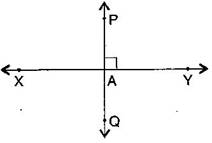∠PAY = 90

3. There are two “set-squares” in your box. What are the measures of the angles that are formed at their corners? Do they have any angle measure that is common?

One set-square has 45,90,45  and other set-square has 60,90,30 . They have 90 as common angle.

4. Study the diagram. The line ll is perpendicular to line m.m.(a) Is CE = EG?
(b)   Does PE bisect CG?
(c) Identify any two line segments for which PE is the perpendicular bisector.
(d) Are these true?
(i) AC > FG
(ii) CD = GH
(iii) BC < EH

(a) Yes, both measure 2 units.
(b) Yes, because CE = EG
(c) DF and CG, BH
(d) (i) True, (ii) True, (iii) True

Exercise 5.6

1. Name the types of following triangles:
(a)Triangle with lengths of sides 7 cm, 8 cm and 9 cm.
(b)ΔABC with AB = 8.7 cm, AC = 7 cm and BC = 6 cm.
(c)ΔPQR such that PQ = QR = PR = 5 cm.
(d)ΔDEF with m∠D = 90
(e)ΔXYZ with m∠Y = 90 and XY = YZ
(f)ΔLMN with m∠L = 30,m∠M = 70 and m∠N = 80.

(a) Scalene triangle
(b) Scalene triangle
(c) Equilateral triangle
(d) Right-angled triangle
(e) Isosceles right-angled triangle

(f) Acute-angled triangle

2. Match the following:
 Measure of Triangle Types of Triangle (i)3 sides of equal length (a) Scalene (ii) 2 sides of equal length (b) Isosceles right angle (iii) All sides are of different length (c) Obtuse angle (iv) 3 acute angles (d) Right angle (v) 1 right angle (e) Equilateral (vi) 1 obtuse angle (f) Acute angle (vii) 1 right angle with two sides of equal length (g) Isosceles

(i) → (e),
(ii) → (g),
(iii) → (a),
(iv) → (f),
(v) → (d),
(vi) → (c),
(vii) → (b)

3. Name each of the following triangles in two different ways: (You may judge the nature of angle by observation)(a) Acute angled triangle and Isosceles triangle
(b) Right-angled triangle and Scalene triangle
(c) Obtuse-angled triangle and Isosceles triangle
(d) Right-angled triangle and Isosceles triangle
(e) Equilateral triangle and acute angled triangle
(f) Obtuse-angled triangle and scalene triangle

4. Try to construct triangles using match sticks. Some are shown here.Can you make a triangle with:
(a) 3 matchsticks?
(b) 4 matchsticks?
(c) 5 matchsticks?
(d) 6 matchsticks?
(Remember you have to use all the available matchsticks in each case)
If you cannot make a triangle, think of reasons for it.

(a) 3 matchsticks
This is an acute angle triangle and it is possible with 3 matchsticks to make a triangle because sum of two sides is greater than third side.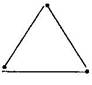(b) 4 matchsticks
This is a square, hence with four matchsticks we cannot make triangle.

(c) 5 matchsticks
This is an acute angle triangle and it is possible to make triangle with five matchsticks, in this case sum of two sides is greater than third side.(d) 6 matchsticks
This is an acute angle triangle and it is possible to make a triangle with the help of 6 matchsticks because sum of two sides is greater than third side.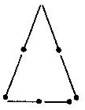Exercise 5.7

1. Say true or false:
(a) Each angle of a rectangle is a right angle.
(b) The opposite sides of a rectangle are equal in length.
(c) The diagonals of a square are perpendicular to one another.
(d) All the sides of a rhombus are of equal length.
(e) All the sides of a parallelogram are of equal length.
(f) The opposite sides of a trapezium are parallel.

(a) True,
(b) True,
(c) True,
(d) True,
(e) False,
(f) False

2. Give reasons for the following:
(a) A square can be thought of as a special rectangle.
(b) A rectangle can be thought of as a special parallelogram.
(c) A square can be thought of as a special rhombus.
(d) Squares, rectangles, parallelograms are all quadrilateral.
(e) Square is also a parallelogram.

(a) Because it's all angles are right angle and opposite sides are equal.
(b) Because its opposite sides are equal and parallel.
(c) Because its four sides are equal and diagonals are perpendicular to each other.
(d) Because all of them have four sides.
(e) Because its opposite sides are equal and parallel.

3. A figure is said to be regular if its sides are equal in length and angles are equal in measure. Can you identify the regular quadrilateral?

A square is a regular quadrilateral.

Exercise 5.8

1. Examine whether the following are polygons. If anyone among these is not, say why?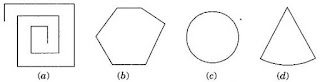(a) As it is not a closed figure, therefore, it is not a polygon.
(b) It is a polygon because it is closed by line segments.
(c) It is not a polygon because it is not made by line segments.
(d) It is not a polygon because it not made only by line segments, it has curved surface also.

2. Name each polygon: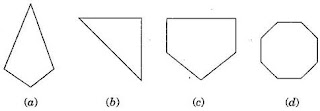(b) Triangle,
(c) Pentagon,
(d) Octagon

3. Draw a rough sketch of a regular hexagon. Connecting any three of its vertices, draw a triangle. Identify the type of the triangle you have drawn.

ABCDEF is a regular hexagon and triangle thus formed by joining AEF is an isosceles triangle.4. Draw a rough sketch of a regular octagon. (Use squared paper if you wish). Draw a rectangle by joining exactly four of the vertices of the octagon.

ABCDEFGH is a regular octagon and CDGH is a rectangle.5. A diagonal is a line segment that joins any two vertices of the polygon and is not a side of the polygon. Draw a rough sketch of a pentagon and draw its diagonals.

ABCDE is the required pentagon and its diagonals are AD, AC, BE and BD.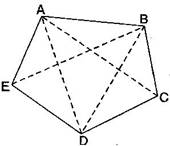Exercise 5.9

1. Match the following:2. What shape is:
(b) A brick?
(c) A match box?

(a) Cuboid
(b) Cuboid
(c) Cuboid
(d) Cylinder
(e) Sphere

## NCERT Solutions for Class 6 Maths Chapter 5 Understanding Elementary Shapes

Understanding Elementary Shapes NCERT Solutions is very essential for scoring good marks in the class test examinations. In this chapter, we will talk about lines and angles, two dimensional figures and also about three dimensional figures.

• A triangle is called a scalene triangle if all the three sides are of unequal length.

• A triangle is said to be an equilateral triangle if the lengths of all of its sides are equal.

• If one of the angles in a triangle is an obtuse angle, then the triangle is called an obtuse-angled triangle.

If you want solution of specific question then you can easily get them from exercisewise NCERT Solutions then you can get them just by clicking on the links given below. It will prepare students to do better during immense pressure and at the same time make them fresh and enhances memory.

• Exercise 5.1 Chapter 5 Class 6 Maths NCERT Solutions
• Exercise 5.2 Chapter 5 Class 6 Maths NCERT Solutions
• Exercise 5.3 Chapter 5 Class 6 Maths NCERT Solutions
• Exercise 5.4 Chapter 5 Class 6 Maths NCERT Solutions
• Exercise 5.5 Chapter 5 Class 6 Maths NCERT Solutions
• Exercise 5.6 Chapter 5 Class 6 Maths NCERT Solutions
• Exercise 5.7 Chapter 5 Class 6 Maths NCERT Solutions
• Exercise 5.8 Chapter 5 Class 6 Maths NCERT Solutions
• Exercise 5.9 Chapter 5 Class 6 Maths NCERT Solutions

These Class 6 NCERT Solutions will help you in gaining more marks in the examinations and prepare for the board exams in better manner. You can study in an organized manner and outperform your classmates.

### NCERT Solutions for Class 6 Maths Chapters:

 Chapter 1 Knowing Our Numbers Chapter 2 Whole Numbers Chapter 3 Playing with Numbers Chapter 4 Basic Geometrical Ideas Chapter 6 Integers Chapter 7 Fractions Chapter 8 Decimals Chapter 9 Data Handling Chapter 10 Mensuration Chapter 11 Algebra Chapter 12 Ratio and Proportion Chapter 13 Symmetry Chapter 14 Practical Geometry

FAQ on Chapter 5 Understanding Elementary Shapes

#### How many exercises in Chapter 5 Understanding Elementary Shapes?

There are total 9 exercises in the chapter 5 which will set a good foundation for your future goals. It will help you in getting a deeper understanding of various topics and improve studying habits. These NCERT Solutions are helpful in grasping the important points inside the chapter.

#### What is a Rhombus?

In a rhombus, all the sides are equal in length, and the opposite sides are parallel to each other. Its diagonals are not equal in length. Also, the opposite angles are equal to each other.

#### What is perpendicular bisector of the line segment?

A bisector of a line segment is a line that divides the line segment into two equal parts. The perpendicular line that divides a line segment into two equal parts is called the perpendicular bisector of the line segment.

#### What do you mean by Parallelogram?

A parallelogram is a four-sided figure in which the opposite sides are parallel to each other and are of equal length. In a parallelogram, the diagonals need not be equal in length.